## NCERT Solutions for Class 9 Science Chapter 4 Structure of the Atom

These Solutions are part of NCERT Solutions for Class 9 Science. Here we have given NCERT Solutions for Class 9 Science Chapter 4 Structure of the Atom. LearnInsta.com provides you the Free PDF download of NCERT Solutions for Class 9 Science (Chemistry) Chapter 4 – Structure of The Atom solved by Expert Teachers as per NCERT (CBSE) Book guidelines. All Chapter 4 – Structure of The Atom Exercise Questions with Solutions to help you to revise complete Syllabus and Score More marks.

More Resources

Practical Based Questions for Class 9 Science Chemistry

NCERT IN TEXT PROBLEMS

IN TEXT QUESTIONS

Question 1.
What are canal rays ?
Canal rays also called anode rays, are seen moving from the anode towards the cathode in the specially designed discharge tube. However, they do not originate from the anode.

Question 2.
If an atom contains one electron and one proton, will it carry any charge or not ?
No, it will not carry any charge because an electron has a unit negative charge and a proton has a unit
positive charge. They neutralise each other.

Question 3.
On the basis of Thomson’s model of an atom explain how the atom is neutral as a whole.
According to Thomson’s Model of an atom, an atom may be regarded as a positively charged sphere in
which protons are present. The negatively charged electrons are believed to be studded or embedded in the sphere. Since the negative charges due to electrons and positive charges due to protons balance each other, the atom as a whole is neutral.

Question 4.
On the basis of Rutherford’s model of an atom, which sub-atomic particle is present in the nucleus of an atom ?
The nucleus of atom is positively charged according to Rutherford’s model of an atom. All the protons in an atom are therefore, present in the nucleus.

Question 5.
Draw a sketch of Bohr’s model of an atom with three shells.Question 6.
What do you think would be the observation if the a-particle scattering experiment is carried out using a foil of a metal other than gold ?
If the metal is a heavy one like gold (e.g. silver, platinum etc.) similar results will be obtained. However if the metal is quite light (e.g. sodium, magnesium etc.), it is just possible that the fast moving and massive α-particles (4u mass) may push the nucleus aside and pass through with slight deviations only.

Question 7.
Name the three sub – atomic particles in an atom.
The sub-atomic particles in an atom are : protons, electrons and neutrons.

Question 8.
Helium atom has atomic mass of 4u and has two protons in its nucleus. How many neutrons does it have ?
Mass number of helium is equal to its atomic mass but has no units.
∴ Mass number (A) of helium = 4
No. of protons in the nucleus = 2 Atomic number (Z) of the element = 2
No. of neutrons (n) = A – Z = 4 – 2 = 2.

Question 9.
Write the distribution of electrons in carbon and sodium atoms.
Carbon(Z = 6) : 2 (K-shell) ; 4(L-shell)
Sodium (Z = 11) : 2 (K-shell) ; 10 (L-shell) ; 1 (M-shell)

Question 10.
If the K and L shells of an atom are full, then what would be the number of electrons in the atom ?
(CBSE 2014)
Maximum no. of electrons in K-shell = 2
Maximum no. of electrons in L-shell = 8
Total no. of electrons in the atom = 2 + 8 = 10

Question 11.
If the number of electrons in an atom is 8 and the number of protons is also 8, then :
(i) What would be the atomic number of the atom ?
(ii) What is the charge on the atom ?
(i) Atomic number (Z) of the atom = No. of protons = No. of electrons = 8
(ii) Charge on the atom = No charge
Actually, when the number of protons and electrons are the same, the atom is neutral.

Question 12.
For the symbols H, D and T, tabulate three sub-atomic particles found in each of them.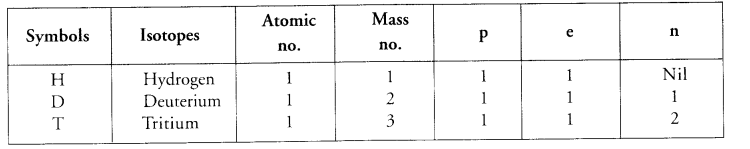Question 13.
Write the electronic configuration of any pair of isotopes and isobars.
(i) Electronic configuration of pair of isotopes :(ii) Electronic configuration of pair of isobars :
Isobars have same mass number but different atomic numbers. For example, elements calcium and argon are isobars :
Ca(Z = 20) : 2, 8, 8, 2
Ar (Z = 18) : 2, 8, 8.
Please note that both the elements have mass number (A) equal to 40.

NCERT END EXERCISE

Question 1.
Compare the properties of electrons, protons and neutrons.Question 2.
What are the limitations of J.J. Thomson’s model of an atom ?
Limitations of Thomson’s model of an atom
(i) It could not explain the result of the scattering experiment performed by Rutherford.
(ii) It did not have any experimental evidence in its support.

Question 3.
What are the limitations of Rutherford’s model of an atom ?
Limitations of Rutherford Model atom

1. Rutherford model of atom could not explain the stability of the atom.
2. Rutherford model of atom could not explain as to how the electrons are distributed in the extra nuclear portion in an atom.

Question 4.

Describe Bohr’s model of an atom.
In 1913, Neils Bohr gave a theory regarding the distribution of electrons in the extra nuclear space in an atom. The main postulates of the theory are listed :

1. In the extra nuclear portion of an atom, the electrons revolve in well defined circular paths known as orbits.
2. These circular orbits are also known as energy levels or energy shells.
3. These have been designated as K, L, M, N, O, … (or as 1, 2, 3, 4, 5, …) based on the energy present.
4. The order of the energy of these energy shells is :
K<L<M<N<0 <…. or 1< 2< 3 < 4<5 <….
5. While revolving in an orbit, the electron is not in a position to either lose or gain energy. In other words, its energy remains stationary. Therefore, these energy states for the electrons are also known as stationary states.

Question 5.
Compare all the proposed models of an atom given in this chapter.
For Thomson’s Model of air atom:

1. It could not explain the result of the scattering experiment performed by Rutherford.
2. It did not have any experimental evidence in its support.

For Rutherford’s Model of an atom:

1. Rutherford model of atom could not explain the stability of the atom.
2. Rutherford model of atom could not explain as to how the electrons are distributed in the extra nuclear portion in an atom.

For Bohr’s Model of air atom:

1. It gave no idea about the shapes of the molecules formed by the combination of atoms.
2. According to Bohr’s theory, an electron in an atom follows a well defined circular path called orbit. However, the later studies have revealed that the path of the electron cannot be followed exactly. It is only of probable nature and not exact as stated by Bohr. However, it is not possible to discuss this concept at the present level of the students.

Question 6.
Summarise the rules for writing the distribution of electrons in various shells for first eighteen elements.
As the electrons have negligible mass, the entire mass of the atom is that of protons and neutrons present in its nucleus. Since each proton and neutron has mass equal to 4 u, therefore, mass of an atom must be the same as the sum of protons and neutrons present in the nucleus. The latter is known as mass number of an element. It is denoted by the symbol A’. The mass number of an element may be defined as :
The sum of the number of protons and neutrons present in the nucleus of its atom.
Mass number (A) = No. of protons + No. of neutrons

Question 7.
Describe valency by taking the examples of silicon and oxygen.
Valency of an element may be defined as the combining capacity of its atom with atoms of other elements in order to acquire 8 electrons (2 in some exceptional cases).
Valency of silicon (Si) : Atomic number of the element is 14. Its electronic distribution is ; K(2), L(8), M(4).
As silicon atom has four valence electrons, it can lose all of them. At the sametime, it can also gain four electrons to have a complete octet. Therefore, the valency of silicon is 4.
Valency of oxygen (O) : Atomic number of the element is 8. Its electronic distribution is ; K(2), L(6)
As oxygen atom has six valence electrons, it needs two more electrons to complete its octet (8-6 = 2). Therefore, valency of oxygen is 2.

Question 8.
Explain with examples
(i) Atomic number
(ii) Mass number
(iii) Isotopes
(iv) Isobars.
(i) Atomic number:
Rutherford stated that the number of unit positive charges present in the nucleus of an atom is known as atomic number of the element It is denoted by the symbol Z.
Atomic no. (Z)= No. ofprotons = No. of electrons
(ii) Mass number:
The mass number of an element may be defined as : the sum of the number of protons and neutrons present in the nucleus of its atom.
Mass number (A) = No. of protons + No. of neutrons
(iii) Isotopes:
Isotopes may be defined as the different atoms of the same element having same atomic number but different mass numbers.(iv) Isobars:
isobars may be defined as the atoms belonging to the different elements with same mass numbers but different atomic numbers.

Question 9.
Na+ ion has completely filled K and L shells. Explain. (CBSE 2013)
Na+ ion is formed when Na atom loses one electron from the valence shell i.e. M shell. The Na+ ion formed has only two shells which are complete
Na : K(2) L(8) M(1) ;
Na+ : K(2) L(8)

Question 10.
If the element bromine is in the form two isotopes which are 7935Br (49.7%) and 8135Br (30.3%), then calculate the average atomic mass of bromine.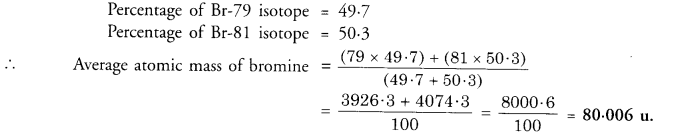Question 11.
The average atomic mass of a sample of element X is 16.2 u. What are the percentages of isotopes 168X and 188X in the sample ? (CBSE 2016)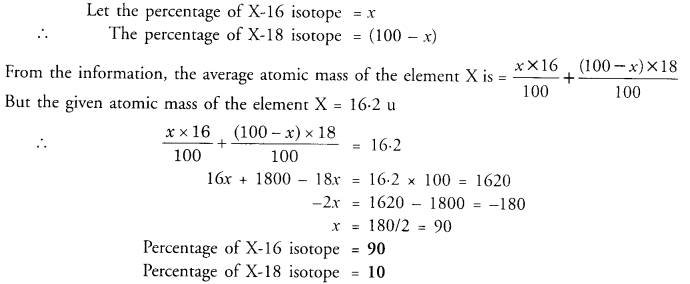Question 12.
If Z = 3, what would be the valency of the element ? Also name the element.
The configuration of the element with Z = 3 is K(2) L(l). It has one electron in the valence shell i.e. L-shell. Its valency is one. The element is lithium (Li).

Question13.
Composition of the nuclei of two atomic species X and Y are given as under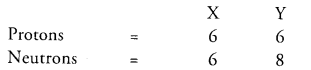Give the mass numbers of X and Y. What is the relation between the two species ?
Mass number of X = protons + neutrons = 6 + 6 =12 Mass number of Y = 6 + 8 = 14
The species X and Y are the isotopes because their atomic numbers are same and their mass numbers are different.

Question 14.
For the following statements, write T for True and F for False.
(a) J.J. Thomson proposed that the nucleus of an atom contains only nucleons.
(b) A neutron is formed by an electron and proton combining together. Therefore, it is neutral.
(c) The mass of an electron is about 1/2000 times that of proton.
(d) An isotope of iodine is used for making tincture of iodine, which is used as medicine.
(a) F
(b) F
(c) T
(d) F.

Question 15.
Rutherford’s alpha-particle scattering experiment was responsible for the discovery of
(a) Atomic Nucleus
(b) Electron
(c) Proton
(d) Neutron.

Question 16.
Isotopes of element have :
(a) the same physical properties
(b) different chemical properties
(c) different number of neutrons
(d) different atomic numbers.
(c) Different number of neutrons.

Question 17.
Number of valence electrons in Cl ion are :
(a) 16
(b) 8
(c) 17
(d) 18.
(d) CE ion has 18 electrons (17 + 1).

Question 18.
Which one of the following is a correct electronic configuration of sodium ?
(a) 2, 8
(b) 8, 2, 1
(c) 2, 1, 8
(d) 2, 8, 1

Question 19.
Complete the following table.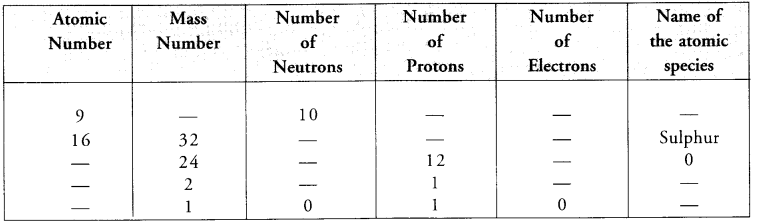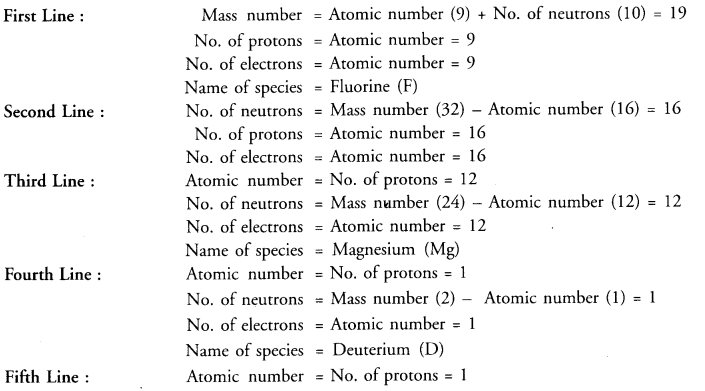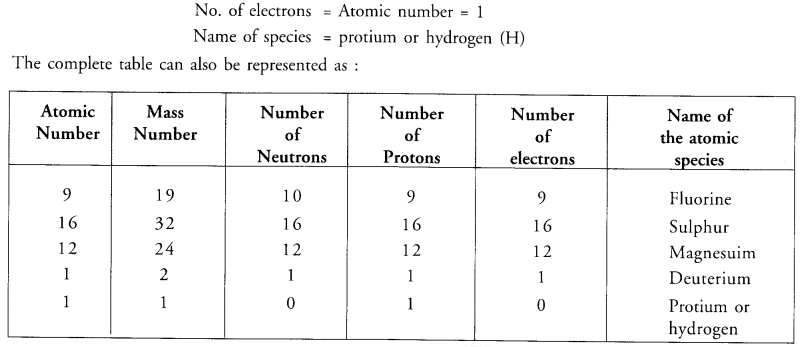Question 1.
Out of the three sub-atomic particles known, which is called universal particle ?
Electron is known as universal particle.

Question 2.
Name a species which has
(i) one proton and one electron
(ii) one proton and two electrons
(i) Protium or hydrogen (H)
(ii) Hydride ion (H).

Question 3.
How many electrons are present in the species He2+ ion ? Suggest another name for it.
He2+ ion has no electrons. It is also called alpha (α) particle.

Question 4.
How many times is radius of extra nuclear protion more than that of the nucleus of an atom ?
Radius of extra nuclear portion is nearly 105 times more as compared to the nucleus.

Question 5.
Is atomic number of an atom always equal to the number of electrons ?
No, it is the case when the atom has no charge. In case of cation (positively charged), atomic number is more than the number of electrons and in case of anion (negatively charged), it is less than the number of electrons.

Question 6.
Out of proton and neutron, which is heavier ?
Neutron is slightly heavier (1.675 x 10-27 kg) than proton (1.67 x 10-27 kg).

Question 7.
Explain why chlorine has relative atomic mass of about 35.5 u ? (CBSE 2016)
Chlorine always exists as two isotopes. These are Cl-35 and Cl-37 isotopes and are present in the ratio of 3:1. The atomic mass of the element is therefore, fractional and is regarded as the relative atomic mass.

Question 8.
Why is proton so named ?
Proton (H+) is formed when hydrogen atom also called protium, loses an electron. Therefore, it is called proton.

Question 9.
The cation is represented as M3+. What is the difference between the number of protons and electrons present ?
In cation M3+, there are three electrons less as compared to protons.

Question 10.
If K and L shells of an atom are completely filled, what will be its name ?
The atom will belong to the noble gas element neon (Ne).

Question 11.
Do isobars have also identical chemical characteristics like isotopes ?
No, these are not identical because the isobars have different atomic numbers as well as different electronic configurations. ‘

Question 12.
The electronic configuration of an element is : 2(K), 8(L), 5(M). Predict its valency.
The valency of the element is 3. It is calculated as : 8 – 5 = 3.

Question 13.
Write two important postulates of Bohr’s model of an atom. (CBSE 2014)
The 2 main postulates of the theory are listed :

1. In the extra nuclear portion of an atom, the electrons revolve in well defined circular paths known as orbits.
2. These circular orbits are also known as energy levels or energy shells.

Question 14.
What is the basic difference between the isotopes of an element ?
Isotopes of an element differ in the number of neutrons leading to different mass numbers.

Question 15.
The element aluminium is written by the symbol fgAI. Write the number of protons, electrons and neutrons present in it.
No. of protons= 13 ; No. of electrons = 13 ; No. of neutrons = 27 – 13 = 14.

Question 16.
What is the number of electrons in the valence shell of chlorine (Z = 17) ?
The electronic distribution of the element is : K(2), L(8), M(7). This means that the valence shell of chlorine has 7 electrons.

Question 17.
Which radioisotope is used for the treatment of cancer ?
Radioisotope Co-60 is used for the treatment of cancer.

Question 18.
Out of C-12 and C-14 isotopes of carbon, which is of radioactive nature ?
C-14 isotope is of radioactive nature.

Question 19.
If an atom has one proton and one electron, state the charge on the atom. Justify your answer. (CBSE 2014)
The atoms is neutral. One unit positive charge on proton cancels with one unit negative charge on electron.

Question 20.
The electronic configuration of an element Z is 2, 8, 6. How many electrons does it require to have a stable
configuration ?
In order to have a stable configuration, the element Z must have 8 electrons in the valence shell. It needs (8 – 6) = 2 electrons for this purpose.

Question 21.
Will 126C and 146C have different valencies ?
Both the species are the isotopes of the same element i.e. the carbon. Since their atomic numbers are same, their electronic configurations as well as valencies will also be the same.

Question 22.
The atom of an element A’ has three electrons in the outermost shell. It loses one of these to the atom of another element ‘B’. What will be the nature and value of charge on the ion which results from ‘A’ ?
Since the atom A’ has lost one electron, the ion formed will be positive and will have +1 charge. It can be represented as A+.

Question 23.
The atom of an element has electronic configuration 2, 8, 6. Does it gain two electrons or lose six electrons to have the configuration of the nearest noble gas element ?
The atom has six electrons in its valence shell. It is very difficult for it to lose six electrons. However, it can gain two electrons from a neighboring atom of another element. By doing so, it will have the electronic configuration of nearest noble gas element argon (2, 8, 8).

Question 24.
Do the elements X and represent pair of isotopes ?
No, they do not because the two elements differ in their atomic numbers. Isotopes have the same atomic
numbers.

Question 25.
An element ‘X’ has mass number 4 and atomic number 2. Find the valency of ‘X’. (CBSE 2012)
The electronic configuration : K(2). Since the K-shell is filled, the valency of ‘X’ = zero.

Question 26.
Name the particles which determine the mass of an atom. (CBSE 2014)
Protons and neutrons present in the nucleus determine the mass of an atom.

Question 27.
What is the number of valence electrons in
(i) Sodium ion (Na+)
(ii) oxide ion (O2-) ? (CBSE 2012)
(i) Sodium ion (Na+) :
No. of electrons : (11 – 1) = 10 ; Electronic configuration = 2, 8.
∴ Na+ ion has 8 valence electrons.
(ii) Oxide ion (O2-) :
No. of electrons : (8 + 2) = 10 ; Electronic configuration = 2, 8
∴ O2- ion has 8 valence electrons.
Both Na+ ion and O2- ion are known as isoelectronic species as they have same number of electrons i.e. 10. Their electronic configuration is similar to that of neon (Z = 10) which is a noble gas element.
This means that O2- ion, Na+ ion and Ne atom are isoelectronic species.

Question 28.
Elements A and ‘B’ have atomic numbers 18 and 16 respectively. Which of these two would be more reactive and why ?
Electronic configuration of A (Z = 18) ; 2, 8, 8
Electronic configuration of B (Z = 16) ; 2, 8, 6
Element A’ has completely filled outermost shell (M-shell). It would be therefore, least reactive.
Element ‘B’ has incomplete outermost shell (M-shell has 6 electrons) only.
Therefore, element ‘B’ would be more reactive than element ‘A’.

Question 29.
Two atoms A and B have the following composition :What are their mass numbers ? What is the relation between the species ?
Mass number of A =17+18 = 35
Mass number of B = 17 + 20 = 37
The atoms A and B represent a pair of isotopes since thery have the same atomic number (17)

Question 30.
The composition of two atomic particles is given :(i) What is the mass number of X ?
(ii) What is the mass number of Y
(iii) What is the relation between X and Y ?
(iv) Which element/elements do they represent ? (CBSE 2012)
(i) Mass number of X = 8 + 8 =16
(ii) Mass number of Y = 8 + 9 = 17
(iii) The atomic particles represent a pair of isotopes.
(iv) The atomic number of the atomic particles is the same as the number of protons (8). The element with atomic number 8 is oxygen.

Question 31.
State whether the following statements are true or false.
(i) One mole of methane has four H atoms.
(ii) The isotopes of an element differ in the number of electrons.
(iii) The elements are identified by their atomic numbers.
(iv) The mass number of an element has specific units.
(v) The K shell of an element cannot have more than eight electrons.
(i) False
(ii) False
(iii) True
(iv) False
(v) False

Question 32.
Name the elements which have the following electronic configuration :
(i) 2, 6
(ii) 2, 7
(iii) 2, 8, 1
(iv) 2, 8, 7
(v) 2, 8
(i) oxygen
(ii) fluorine
(iii) sodium
(iv) chlorine
(v) neon.

Question 33.
Give three points of distinction between isotopes and isobars.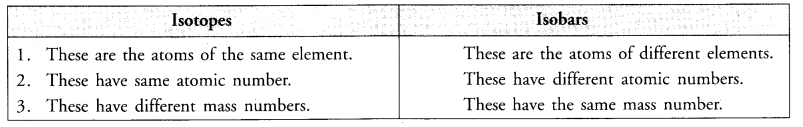Question 34.
The details about three atoms X, Y and Z are given. Give information about their atomic number, mass number and valency ?Question 35.
Why do elements helium, neon and argon have zero valencies ?
The electronic configuration of three elements are given :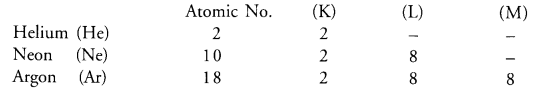The first element helium (He) has only one shell and has maximum of two electrons in the only shell (K-shell) which it has.
Similarly, the next two elements neon (Ne) and argon (Ar) also have the maximum of eight electrons in their outermost shells L and M respectively.
This means that the atoms of all the three elements donot have any urge to either lose or gain electrons. Therefore, they show zero valencies. These are also called noble gas or inert gas elements as they donot take part in the chemical combination.

Question 36.
Give the electronic distribution in magnesium atom and magnesium ion. How do these electronic configuration differ ?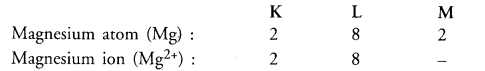Mg2+ ion has only two shells and its electronic configuration is similar to that of noble gas element neon (Ne). Mg atom has three shells with 2 electrons in the outer shell i.e. M-shell.

Question 37.
(a) Give the schematic atomic structures of chlorine atom and chloride ion.
(b) Mention two uses of isotopes in the field of medicine.
(c) How are the following pair of elements known as ?
4018Ar,   4020Ca
(a)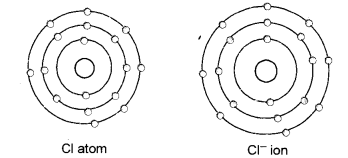(b) (i) Radioisotope 1-131 is used to check the working of thyroid gland.
(ii) Radioisotope Co – 60 is used in cancer therapy.
(c) These are known as isobars. Since they have the same mass number but different atomic numbers.

Question 38.
Answer the following in one line or two :
(a) What is the maximum number of electrons that can be accomodated in the outermost energy shell in an atom ?
(b) On the basis of Thomsons model of an atom, explain how an atom is neutral as a whole.
(c) How many neutrons are present in hydrogen atom ?
(d) Do isobars belong to the same element ?
(e) An element has five electrons in the M-shell which is the outermost shell. Write its electronic configuration.
(CBSE 2011)
(a) The outermost energy shell in an atom can have a maximum of eight electrons.
(b) According to Thomson’s model of an atoms, all the protons in an atom are present in the positively charged sphere while negatively charged electrons are studded in this sphere. Since the electrons and protons are equal in number each carrying one unit charge, the atom as a whole is electrically neutral.
(c) Hydrogen atom has no neutron.
(d) No, isolars belong to different elements since they differ in their atomic numbers.
(e) The electronic configuration of the element is K(2) L(8) M(5).

Question 39.
Which observations in scattering experiment led Rutherford to make the following conclusions :
(i) Most of the space in an atom is empty.
(ii) Whole mass of an atom is present in its centre.
(iii) Nucleus is positively charged.
(i) As most of the alpha particles passed through undeflected, this means that they did not come across any obstruction in their path. Thus, most of the space in an atom is expected to be empty.
(ii)

1. As a few alpha particles suffered minor deflections and a very few major deflections, this means that these must have met with some obstructions in their path.
2. This obstruction must be :
1. Very small : Only a few particles were obstructed by it.Massive : Each alpha particle has 4u mass and is quite heavy.
2. It could easily pass through a light obstruction by pushing it aside.

(iii) Positively charged : Alpha particles have positive charge. Since they were repelled or deflected back, the obstruction must also carry same charge i.e., positive charge, (similarly charged particles always repel each other

Question 40.

With the help of suitable activities shows that
(i) Cathode rays travel in straight line
(ii) Cathode rays consist of material particles. (CBSE 2012)
(i) Cathode rays always travel in straight line. In order to demonstrate this, place an object in the path of the cathode rays that are emitted in a discharge tube. The shadow of the object will immediately appear behind it. This shows that these rays are stopped by the object and thus, travel in straight line.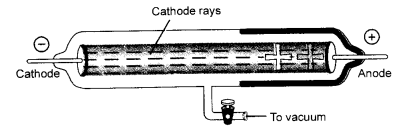(ii) Cathode rays consist of negatively charged particles known as electrons which have definite mass. In order to demonstrate this, place a light paddle wheel of mica mounted on an axle in the path of cathode rays. It will start rotating. This shows that the cathode rays or electrons are material particles.Question 41.
How did Bohr distribute electrons in different shells in an atom ?
In 1913, Neils Bohr gave a theory regarding the distribution of electrons in the extra nuclear space in an atom. The main postulates of the theory are listed :

1. In the extra nuclear portion of an atom, the electrons revolve in well defined circular paths known as orbits.
2. These circular orbits are also known as energy levels or energy shells.
3. These have been designated as K, L, M, N, O, … (or as 1, 2, 3, 4, 5, …) based on the energy present.
4. The order of the energy of these energy shells is :
K<L<M<N<0 <…. or 1< 2< 3 < 4<5 <….
5. While revolving in an orbit, the electron is not in a position to either lose or gain energy. In other words, its energy remains stationary. Therefore, these energy states for the electrons are also known as stationary states

Question 42.
The average atomic mass of a sample of an element ‘X’ is 16.2 u. What is the percentage of each isotope 168X and 188X in the sample ?
Let the percentage of  168X isotope = x
The percentage of  188X isotope = 100 – x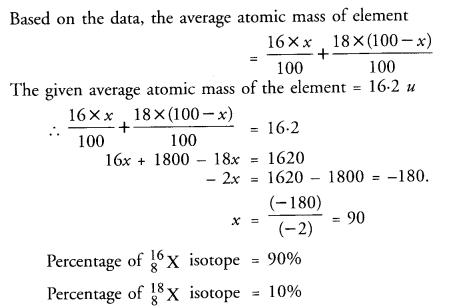Question 43.
Give reasons for the following :
(a) Isotopes of an element are chemically similar.
(b) An atom is electrically neutral.
(c) Noble gases show least reactivity
(d) Nucleus of an atom is heavy and and positively charged.
(e) Ions are more stable than atoms.
(a) Isotopes of an element have same atomic number as well as electronic distribution. Since the chemical properties of the elements are related to their electronic distribution or configuration, the elements with similar configuration have similar properties. Thus, the isotopes of an element are chemically similar.
(b) In an atom, the number of protons in the nucleus is the equal to the number of electrons in the extra nuclear portion. Since each proton and each electron has the same charge but with opposite magnitude, atom is therefore, electrically neutral.
(c) The atoms of the noble gas elements have complete outermost electron shells. With the exception of helium (He) which has two electrons in its only shell (K-shell), the rest of the members of the family have eight electrons in their outermost or valence shell. This means that atoms of these elements have no urge to take part in chemical combination or they are least reactive in nature.
(d) Positively charged : Alpha particles have positive charge. Since they were repelled or deflected back, the obstruction must also carry same charge i.e., positive charge, (similarly charged particles always repel each other
(e) When an atom changes into ion (cation or anion) the valence shell of the ion has a complete octet, (i.e. it has eight electrons in the outermost or valence shell). In some ions, the valence shell is K-shell and it has only two electrons (e.g. Li+ ion or H ion). The ions are therefore, quite stable in nature.

Question 44.
The following data represents the distribution of electrons, protons and neutrons in atoms of four elements . A, B, C, D.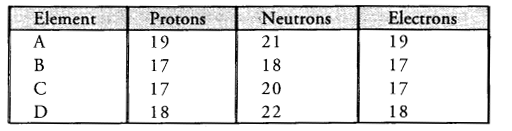(a) Describe the electronic distribution in atom of element B.
(b) Is element B a metal or a non-metal ? Why ?
(c) Which two elements form a pair of Isotopes ?
(d) Which two elements form in pair of Isobars ?